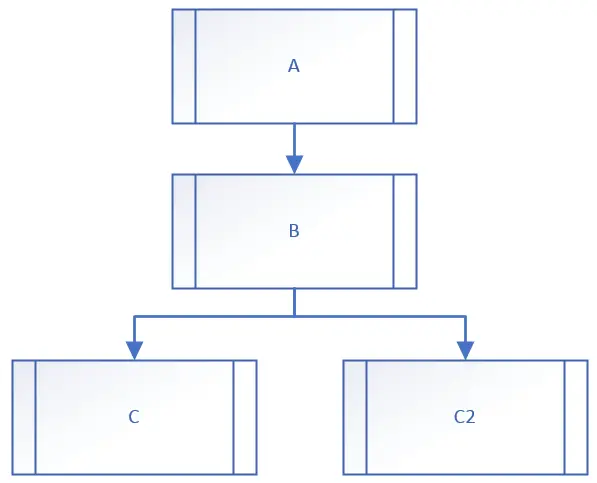# Python super() metod

There are a lot of content out there explaining and showing examples of how to use Python’s super() method:

Still, after reading all of them, I wasn’t exactly sure which attribute to pass to the super() method in order to invoke a method from a certain class on the inheritance chain.

## Example

Let’s imagine the following inheritance tree.python super() method example

The code for classes A, B and C follows:

``````class A:
def __init__(self):
print("A initiated")

class B(A):
def __init__(self):
super().__init__()
print("B initiated")

class C(B):
def __init__(self):
super(C, self).__init__()
print("C initiated")
``````

As it’s seen from the code above, all classes overload their direct parents’ `__init__()` method. Therefore, it suffices to use super() without any attributes. So, if I run `c = C()`, I get the following output:

``````A initiated
B initiated
C initiated
``````

Now, let’s imagine we would like the class C2 to jump B's `__init__()` method, but we would like to run A's `__init__()` method. In other words, we would like to inherit the `__init__()` method from A, instead of B, even though our class' direct parent is B. That's where class C2 comes into play. Let's see its implementation.

``````class C2(B):
def __init__(self):
super(B, self).__init__()
print("other C initiated")
``````

As it can be seen from the code above, this time we are passing attributes to the super() method in order to choose exactly which super class to choose when invoking the method. This time, when I run c2 = C2(), this is the output:

``````A initiated
other C initiated
``````

We have effectively "jumped" the direct parent `__init__()` and instead A's `__init__()` method was invoked only.

# SUBSCRIBE FOR NEW ARTICLES